# # d3-shape

`D3` 的其他特性一样，这些图形也是又数据驱动的: 每个图形生成器都暴露了一个如何将数据映射到可视化表现的访问器。例如你可以通过 scaling (opens new window) 定义一个时间序列的线条生成器以生成图表:

``````var line = d3.line()
.x(function(d) { return x(d.date); })
.y(function(d) { return y(d.value); });
``````

``````path.datum(data).attr("d", line);
``````

``````line.context(context)(data);
``````

## # Installing

`NPM` 安装: `npm install d3-shape`. 也可以下载 latest release (opens new window). 此外还可以从 d3js.org (opens new window)standalone library (opens new window) 或作为 D3 4.0 (opens new window) 的一部分载入. 支持 `AMD`, `CommonJS` 以及基本的标签引入形式，如果使用标签引入则会暴露全局 `d3` 变量:

``````<script src="https://d3js.org/d3-path.v1.min.js"></script>
<script src="https://d3js.org/d3-shape.v1.min.js"></script>
<script>

var line = d3.line();

</script>
``````

## # Arcs

`arc` 生成器用来在饼图或圆环图中生成 circular(圆形) (opens new window)annular(环形) (opens new window) 扇形。如果 startend 之间的角度(angular span)差大于 τ (opens new window)`arc` 生成器将会产生一个完整的圆或环。如果小于 `τ` 则生成的扇形可能有 rounded corners(圆角)angular padding(角度间隙)。弧的中心总是在 ⟨0,0⟩; 可以使用 `transform` (参考 SVG (opens new window), Canvas (opens new window)) 来将其移动到指定的位置。

# d3.arc() <源码> (opens new window)

# arc(arguments…) <源码> (opens new window)

``````var arc = d3.arc();

arc({
startAngle: 0,
endAngle: Math.PI / 2
}); // "M0,-100A100,100,0,0,1,100,0L0,0Z"
``````

``````var arc = d3.arc()
.startAngle(0)
.endAngle(Math.PI / 2);

arc(); // "M0,-100A100,100,0,0,1,100,0L0,0Z"
``````

# arc.centroid(arguments…) <源码> (opens new window)

``````function innerRadius(d) {
}
``````

``````function outerRadius(d) {
}
``````

``````function cornerRadius() {
return 0;
}
``````

# arc.startAngle([angle]) <源码> (opens new window)

``````function startAngle(d) {
return d.startAngle;
}
``````

angle 以弧度的形式指定，`0` 表示 `12` 点钟方向并且顺时针方向为正。如果 |`endAngle` - `startAngle`| ≥ `τ` 则会绘制一个完整的扇形或圆环。

# arc.endAngle([angle]) <源码> (opens new window)

``````function endAngle(d) {
return d.endAngle;
}
``````

angle 以弧度的形式指定，`0` 表示 `12` 点钟方向并且顺时针方向为正。如果 |`endAngle` - `startAngle`| ≥ `τ` 则会绘制一个完整的扇形或圆环。

# arc.padAngle([angle]) <源码> (opens new window)

``````function padAngle() {
}
``````

# arc.context([context]) <源码> (opens new window)

## # Pies

`pie` 生成器不会直接生成图形，但是会计算生成饼图或环形图所需要的角度信息，这些角度信息可以被传递给 arc generator

# d3.pie() <源码> (opens new window)

# pie(data[, arguments…]) <源码> (opens new window)

• `data` - 输入数据; 对应输入数组中的数据元素.
• `value` - `arc` 对应的 value.
• `index` - `arc` 基于 `0`sorted index(排序后的索引).
• `startAngle` - `arc`start angle.
• `endAngle` - `arc`end angle.
• `padAngle` - `arc`pad angle.

``````var data = [1, 1, 2, 3, 5, 8, 13, 21];
var arcs = d3.pie()(data);
``````

`pie()` constructs(构造) 一个默认的 `pie` 生成器。`pie()(data)` 为指定的数据集 invokes(调用) 饼图生成器，返回一组对象数组:

``````[
{"data":  1, "value":  1, "index": 6, "startAngle": 6.050474740247008, "endAngle": 6.166830023713296, "padAngle": 0},
{"data":  1, "value":  1, "index": 7, "startAngle": 6.166830023713296, "endAngle": 6.283185307179584, "padAngle": 0},
{"data":  2, "value":  2, "index": 5, "startAngle": 5.817764173314431, "endAngle": 6.050474740247008, "padAngle": 0},
{"data":  3, "value":  3, "index": 4, "startAngle": 5.468698322915565, "endAngle": 5.817764173314431, "padAngle": 0},
{"data":  5, "value":  5, "index": 3, "startAngle": 4.886921905584122, "endAngle": 5.468698322915565, "padAngle": 0},
{"data":  8, "value":  8, "index": 2, "startAngle": 3.956079637853813, "endAngle": 4.886921905584122, "padAngle": 0},
{"data": 13, "value": 13, "index": 1, "startAngle": 2.443460952792061, "endAngle": 3.956079637853813, "padAngle": 0},
{"data": 21, "value": 21, "index": 0, "startAngle": 0.000000000000000, "endAngle": 2.443460952792061, "padAngle": 0}
]
``````

# pie.value([value]) <源码> (opens new window)

``````function value(d) {
return d;
}
``````

``````var data = [
{"number":  4, "name": "Locke"},
{"number":  8, "name": "Reyes"},
{"number": 15, "name": "Ford"},
{"number": 16, "name": "Jarrah"},
{"number": 23, "name": "Shephard"},
{"number": 42, "name": "Kwon"}
];

var arcs = d3.pie()
.value(function(d) { return d.number; })
(data);
``````

``````var arcs = d3.pie()(data.map(function(d) { return d.number; }));
``````

# pie.sort([compare]) <源码> (opens new window)

compare 函数会传递两个参数 ab, 每个元素都来自输入数据。如果数据 a 对应的扇形在 b 前面，则比较函数应该返回小于 `0` 的值; 如果 a 对应的扇形在 b 的后面则比较函数应返回大于 `0` 的值;返回 `0` 表示 ab 的相对位置不做任何调整。例如根据 `name` 对生成的扇形数组进行排序:

``````pie.sort(function(a, b) { return a.name.localeCompare(b.name); });
``````

# pie.sortValues([compare]) <源码> (opens new window)

``````function compare(a, b) {
return b - a;
}
``````

``````pie.sortValues(function(a, b) { return a - b; });
``````

# pie.startAngle([angle]) <源码> (opens new window)

``````function startAngle() {
return 0;
}
``````

# pie.endAngle([angle]) <源码> (opens new window)

``````function endAngle() {
return 2 * Math.PI;
}
``````

# pie.padAngle([angle]) <源码> (opens new window)

``````function padAngle() {
return 0;
}
``````

## # Lines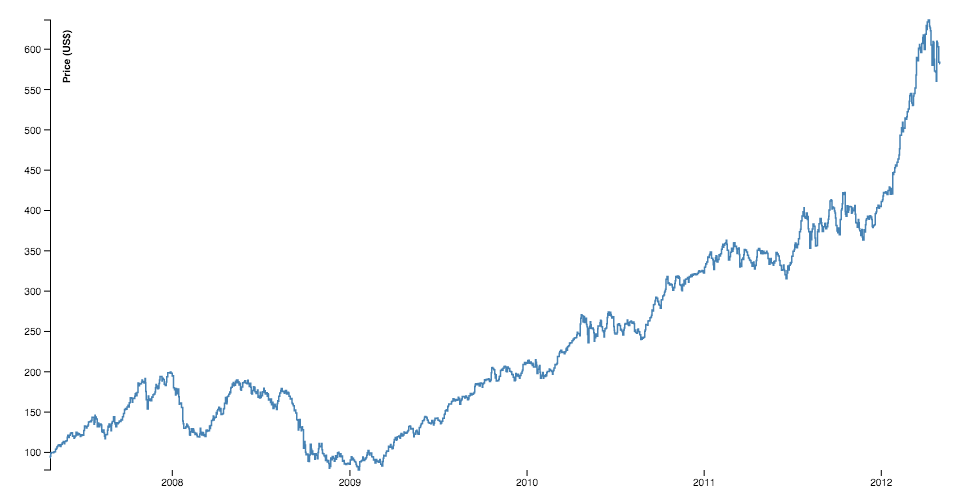(opens new window)

`line` 生成器可以用来生成线条图需要的 spline (opens new window)polyline (opens new window)。线条也可以被用在其他的可视化类型中，比如 hierarchical edge bundling (opens new window)

# d3.line() <源码> (opens new window)

Constructs a new line generator with the default settings.

# line(data) <源码> (opens new window)

# line.x([x]) <源码> (opens new window)

``````function x(d) {
return d;
}
``````

``````var data = [
{date: new Date(2007, 3, 24), value: 93.24},
{date: new Date(2007, 3, 25), value: 95.35},
{date: new Date(2007, 3, 26), value: 98.84},
{date: new Date(2007, 3, 27), value: 99.92},
{date: new Date(2007, 3, 30), value: 99.80},
{date: new Date(2007, 4,  1), value: 99.47},
…
];

var line = d3.line()
.x(function(d) { return x(d.date); })
.y(function(d) { return y(d.value); });
``````

# line.y([y]) <源码> (opens new window)

``````function y(d) {
return d;
}
``````

# line.defined([defined]) <源码> (opens new window)

``````function defined() {
return true;
}
``````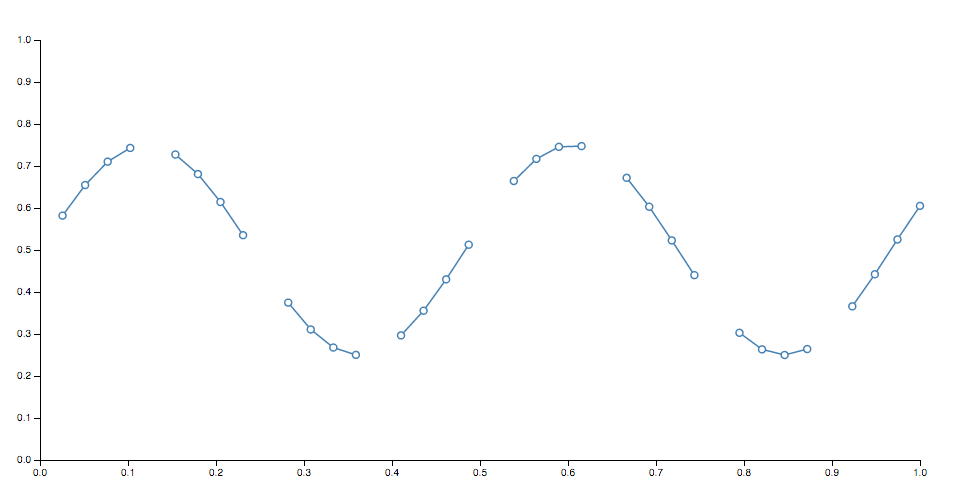(opens new window)

# line.curve([curve]) <源码> (opens new window)

# line.context([context]) <源码> (opens new window)

# d3.lineRadial() <源码> (opens new window)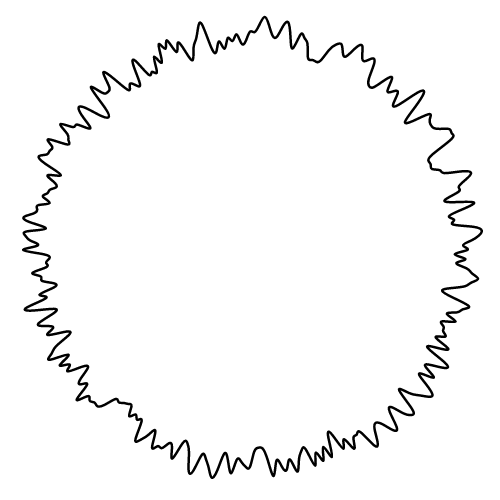# lineRadial(data) <源码> (opens new window)

# lineRadial.angle([angle]) <源码> (opens new window)

# lineRadial.curve([curve]) <源码> (opens new window)

## # Areas

`area generator(区域生成器)` 用来在 `area` 图中生成区域图。一个区域图由两条边界 lines 定义，可以是曲线或折线。通常情况下两条边界线共享一个 x-values (x0 = x1)，仅仅是 y-value (y0y1) 不一样。大多数情况下，`y0` 会被定义为一个常量 zero (opens new window). 第一条线 (也就是 上侧线) 由 `x1``y1` 定义渲染。而第二条线 (也就是 基线) 由 `x0``y0` 定义渲染。有了 curveLinear curve，就可以生成一个顺时针方向的多边形。

# d3.area() <源码> (opens new window)

# area(data) <源码> (opens new window)

# area.x([x]) <源码> (opens new window)

# area.x0([x]) <源码> (opens new window)

``````function x(d) {
return d;
}
``````

``````var data = [
{date: new Date(2007, 3, 24), value: 93.24},
{date: new Date(2007, 3, 25), value: 95.35},
{date: new Date(2007, 3, 26), value: 98.84},
{date: new Date(2007, 3, 27), value: 99.92},
{date: new Date(2007, 3, 30), value: 99.80},
{date: new Date(2007, 4,  1), value: 99.47},
…
];

var area = d3.area()
.x(function(d) { return x(d.date); })
.y1(function(d) { return y(d.value); })
.y0(y(0));
``````

# area.x1([x]) <源码> (opens new window)

# area.y([y]) <源码> (opens new window)

# area.y0([y]) <源码> (opens new window)

``````function y() {
return 0;
}
``````

# area.y1([y]) <源码> (opens new window)

``````function y(d) {
return d;
}
``````

# area.defined([defined]) <源码> (opens new window)

``````function defined() {
return true;
}
``````(opens new window)

# area.curve([curve]) <源码> (opens new window)

# area.context([context]) <源码> (opens new window)

# area.lineX0() <源码> (opens new window)
# area.lineY0() <源码> (opens new window)

# area.lineX1() <源码> (opens new window)

# area.lineY1() <源码> (opens new window)

# d3.areaRadial() <源码> (opens new window)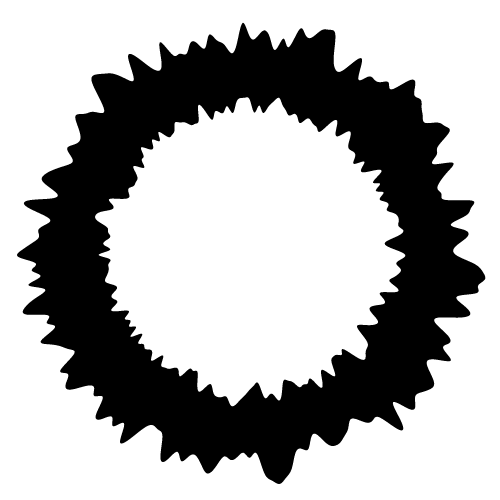# areaRadial.angle([angle]) <源码> (opens new window)

# areaRadial.startAngle([angle]) <源码> (opens new window)

# areaRadial.endAngle([angle]) <源码> (opens new window)

# areaRadial.curve([curve]) <源码> (opens new window)

# areaRadial.lineStartAngle() <源码> (opens new window)

# areaRadial.lineEndAngle() <源码> (opens new window)

## # Curves

lines 被定义为一系列二维点 [x, y]，areas 类似的可以由顶线和基线定义，但是还有一个任务就是把这些离散的点转换为连续的线条: 例如如何在这些点之间进行插值，插值的方式有很多种。

``````var line = d3.line()
.x(function(d) { return x(d.date); })
.y(function(d) { return y(d.value); })
.curve(d3.curveCatmullRom.alpha(0.5));
``````

# d3.curveBasis(context) <源码> (opens new window)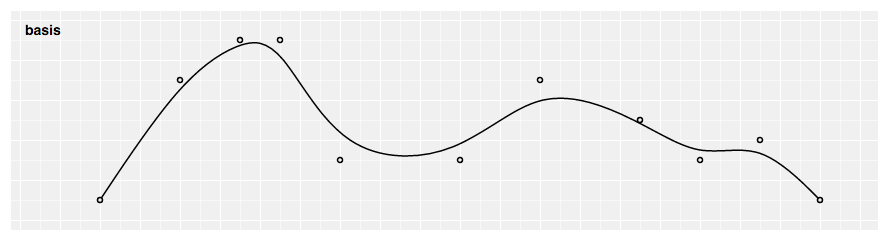# d3.curveBasisClosed(context) <源码> (opens new window)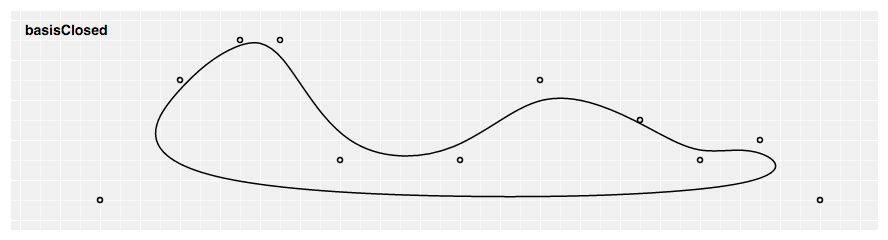# d3.curveBasisOpen(context) <源码> (opens new window)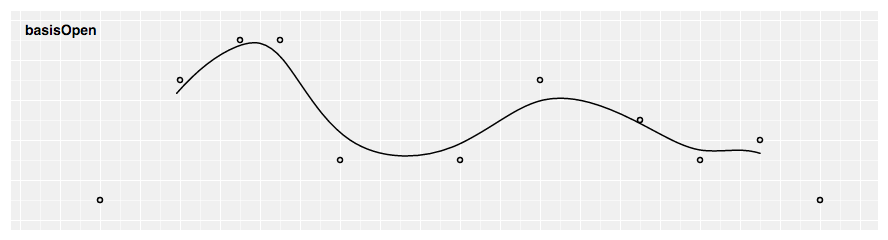# d3.curveBundle(context) <源码> (opens new window)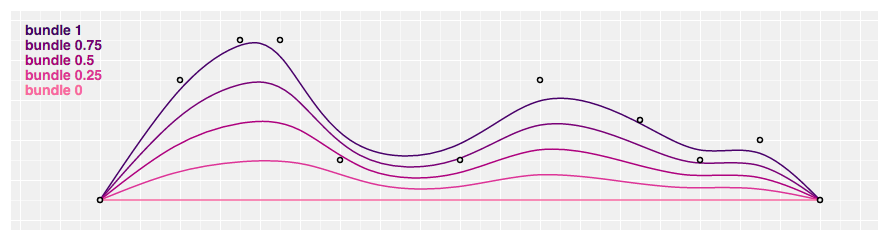# bundle.beta(beta) <源码> (opens new window)

``````var line = d3.line().curve(d3.curveBundle.beta(0.5));
``````

# d3.curveCardinal(context) <源码> (opens new window)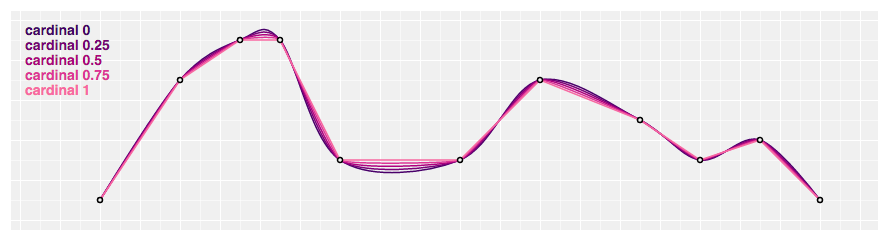# d3.curveCardinalClosed(context) <源码> (opens new window)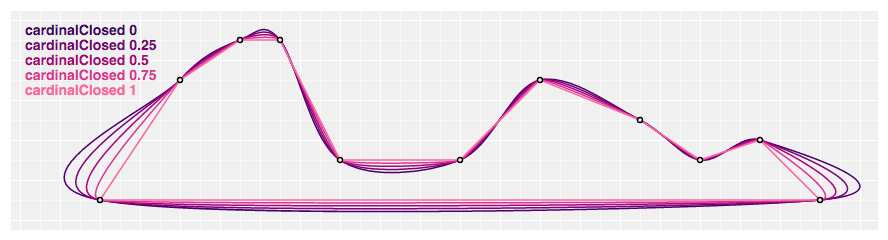# d3.curveCardinalOpen(context) <源码> (opens new window)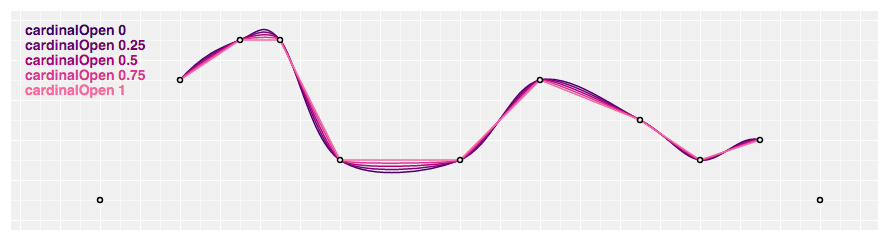# cardinal.tension(tension) <源码> (opens new window)

``````var line = d3.line().curve(d3.curveCardinal.tension(0.5));
``````

# d3.curveCatmullRom(context) <源码> (opens new window)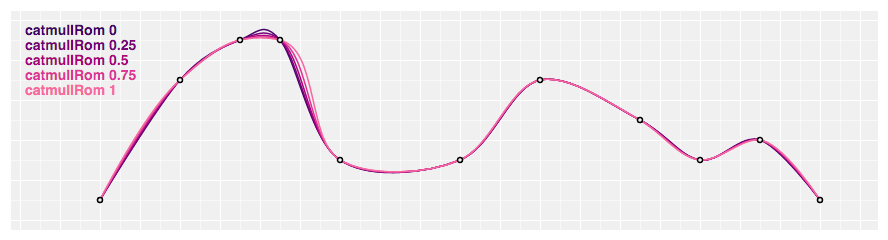# d3.curveCatmullRomClosed(context) <源码> (opens new window)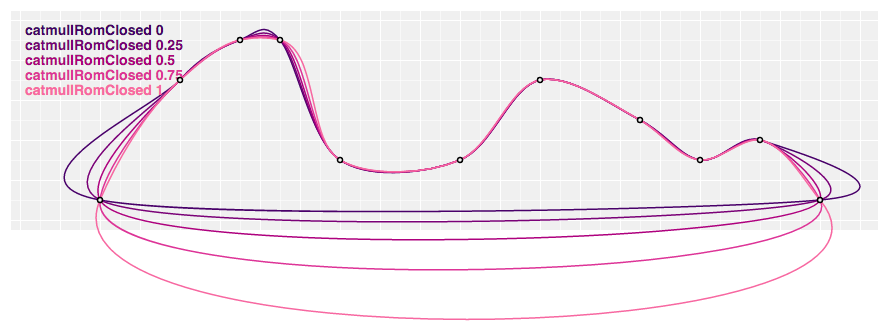# d3.curveCatmullRomOpen(context) <源码> (opens new window)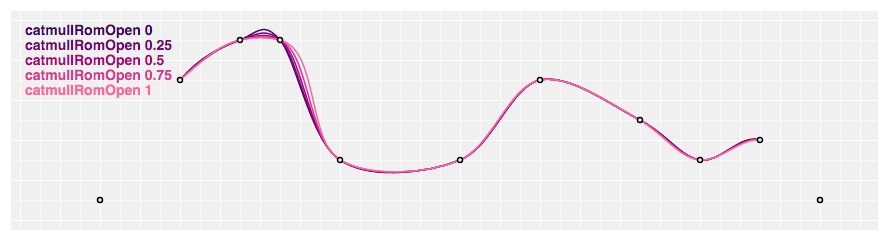# catmullRom.alpha(alpha) <源码> (opens new window)

``````var line = d3.line().curve(d3.curveCatmullRom.alpha(0.5));
``````

# d3.curveLinear(context) <源码> (opens new window)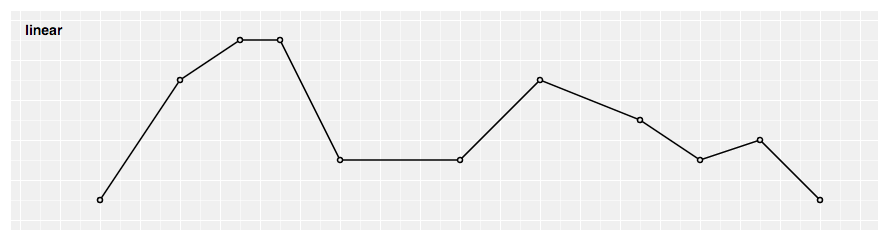# d3.curveLinearClosed(context) <源码> (opens new window)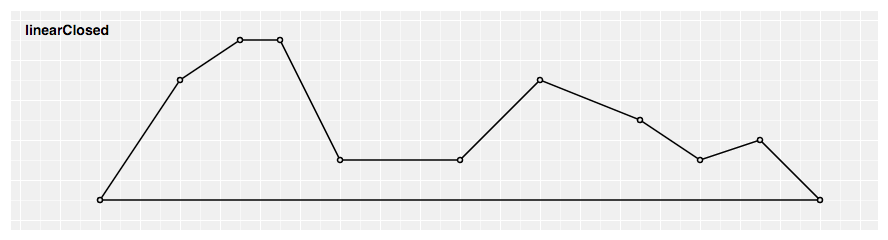# d3.curveMonotoneX(context) <源码> (opens new window)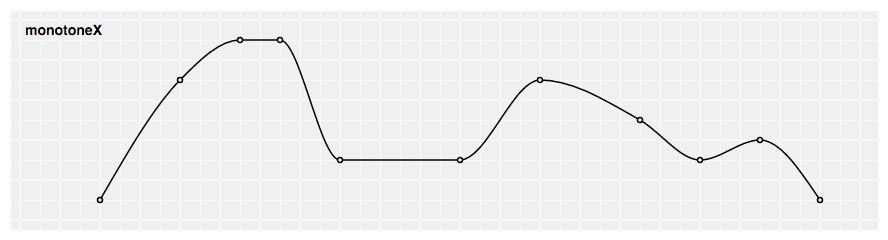# d3.curveMonotoneY(context) <源码> (opens new window)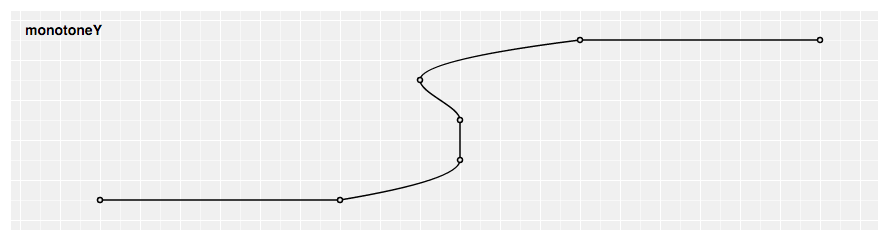# d3.curveNatural(context) <源码> (opens new window)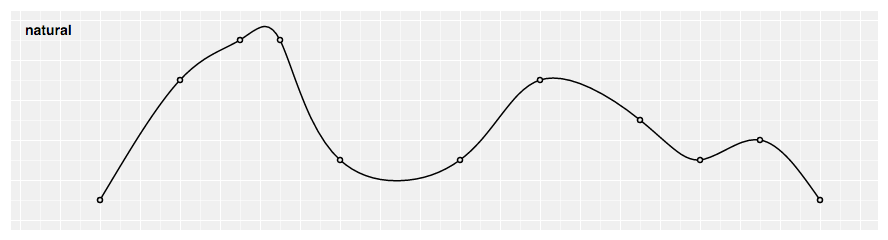# d3.curveStep(context) <源码> (opens new window)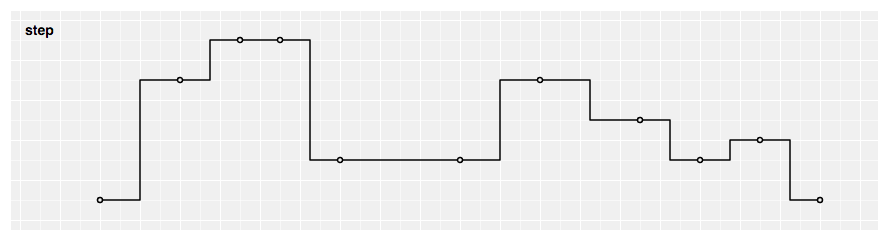# d3.curveStepAfter(context) <源码> (opens new window)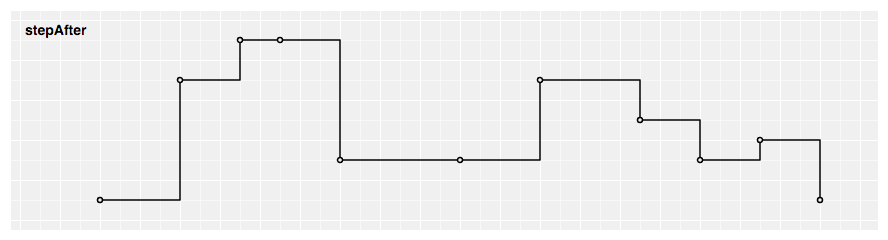# d3.curveStepBefore(context) <源码> (opens new window)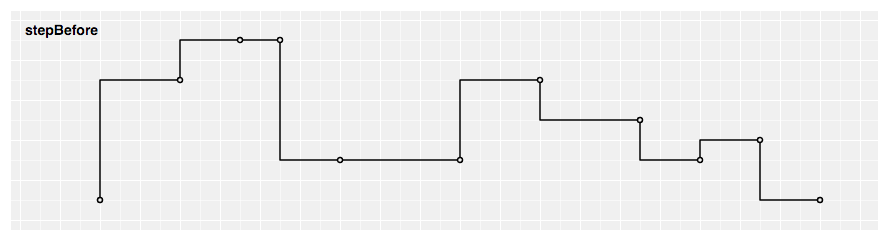## # Custom Curves

`Curves` 通常不会直接使用，而是传递给 line.curvearea.curve. 但是，如果你对内置的曲线不满意的话可以使用如下接口定义自己的曲线实现来替代内置的曲线。你也可以使用这个带有内置曲线类型的低级接口作为线和面积生成器的替代选择。

# curve.areaStart() <源码> (opens new window)

# curve.areaEnd() <源码> (opens new window)

# curve.lineStart() <源码> (opens new window)

# curve.lineEnd() <源码> (opens new window)

# curve.point(x, y) <源码> (opens new window)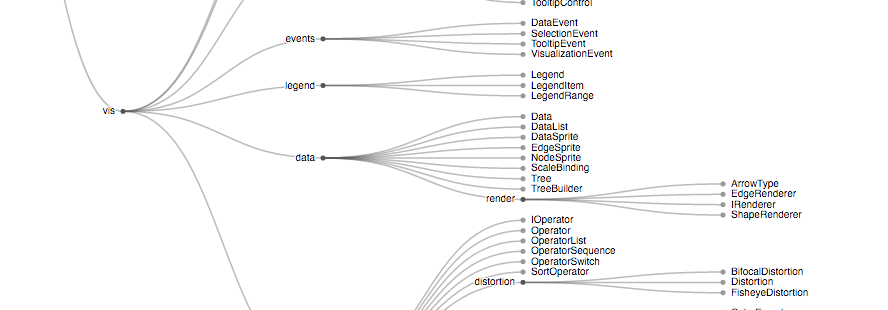(opens new window)

`link` 用来生成从一个源点到目标点的光滑的三次贝塞尔曲线。曲线在起点和终点的切线要么是 vertical，要么是 horizontal，要么是 radial的。

# d3.linkVertical() <源码> (opens new window)

``````var link = d3.linkVertical()
.x(function(d) { return d.x; })
.y(function(d) { return d.y; });
``````

# d3.linkHorizontal() <源码> (opens new window)

``````var link = d3.linkHorizontal()
.x(function(d) { return d.y; })
.y(function(d) { return d.x; });
``````

# link(arguments…) <源码> (opens new window)

``````link({
source: [100, 100],
target: [300, 300]
});
``````

# link.source([source]) <源码> (opens new window)

``````function source(d) {
return d.source;
}
``````

# link.target([target]) <源码> (opens new window)

``````function target(d) {
return d.target;
}
``````

# link.x([x]) <源码> (opens new window)

``````function x(d) {
return d;
}
``````

# link.y([y]) <源码> (opens new window)

``````function y(d) {
return d;
}
``````

# link.context([context]) <源码> (opens new window)

``````var link = d3.linkRadial()
.angle(function(d) { return d.x; })
``````

## # Symbols

# d3.symbol() <源码> (opens new window)

# symbol(arguments…) <源码> (opens new window)

# symbol.type([type]) <源码> (opens new window)

``````function type() {
return circle;
}
``````

# symbol.size([size]) <源码> (opens new window)

``````function size() {
return 64;
}
``````

# symbol.context([context]) <源码> (opens new window)

# d3.symbols

# d3.symbolCircle <源码> (opens new window)

# d3.symbolCross <源码> (opens new window)

# d3.symbolDiamond <源码> (opens new window)

# d3.symbolSquare <源码> (opens new window)

# d3.symbolStar <源码> (opens new window)

# d3.symbolTriangle <源码> (opens new window)

# d3.symbolWye <源码> (opens new window)

`Y` 形符号

## # Custom Symbol Types

# symbolType.draw(context, size)

## # Stacks

pie generator 类似，堆叠布局生成器不会直接产生形状。它是用来计算堆叠数据的，然后传给 area generator 或者直接使用(定位条形图)。

# d3.stack() <源码> (opens new window)

# stack(data[, arguments…]) <源码> (opens new window)

Generates a stack for the given array of data, returning an array representing each series. Any additional arguments are arbitrary; they are simply propagated to accessors along with the `this` object.

Month Apples Bananas Cherries Dates
1/2015 3840 1920 960 400
2/2015 1600 1440 960 400
3/2015 640 960 640 400
4/2015 320 480 640 400

`JavaScript` 中可以表示为对象：

``````var data = [
{month: new Date(2015, 0, 1), apples: 3840, bananas: 1920, cherries: 960, dates: 400},
{month: new Date(2015, 1, 1), apples: 1600, bananas: 1440, cherries: 960, dates: 400},
{month: new Date(2015, 2, 1), apples:  640, bananas:  960, cherries: 640, dates: 400},
{month: new Date(2015, 3, 1), apples:  320, bananas:  480, cherries: 640, dates: 400}
];
``````

``````var stack = d3.stack()
.keys(["apples", "bananas", "cherries", "dates"])
.order(d3.stackOrderNone)
.offset(d3.stackOffsetNone);

var series = stack(data);
``````

``````[
[[   0, 3840], [   0, 1600], [   0,  640], [   0,  320]], // apples
[[3840, 5760], [1600, 3040], [ 640, 1600], [ 320,  800]], // bananas
[[5760, 6720], [3040, 4000], [1600, 2240], [ 800, 1440]], // cherries
[[6720, 7120], [4000, 4400], [2240, 2640], [1440, 1840]], // dates
]
``````

# stack.keys([keys]) <源码> (opens new window)

# stack.value([value]) <源码> (opens new window)

``````function value(d, key) {
return d[key];
}
``````

# stack.order([order]) <源码> (opens new window)

``````function orderNone(series) {
var n = series.length, o = new Array(n);
while (--n >= 0) o[n] = n;
return o;
}
``````

# stack.offset([offset]) <源码> (opens new window)

``````function offsetNone(series, order) {
if (!((n = series.length) > 1)) return;
for (var i = 1, s0, s1 = series[order], n, m = s1.length; i < n; ++i) {
s0 = s1, s1 = series[order[i]];
for (var j = 0; j < m; ++j) {
s1[j] += s1[j] = s0[j];
}
}
}
``````

## # Stack Orders

# d3.stackOrderAscending(series) <源码> (opens new window)

# d3.stackOrderDescending(series) <源码> (opens new window)

# d3.stackOrderInsideOut(series) <源码> (opens new window)

# d3.stackOrderNone(series) <源码> (opens new window)

# d3.stackOrderReverse(series) <源码> (opens new window)

## # Stack Offsets

# d3.stackOffsetExpand(series, order) <源码> (opens new window)

# d3.stackOffsetDiverging(series, order) <源码> (opens new window)

# d3.stackOffsetNone(series, order) <源码> (opens new window)

`0` 为基线。

# d3.stackOffsetSilhouette(series, order) <源码> (opens new window)

# d3.stackOffsetWiggle(series, order) <源码> (opens new window)